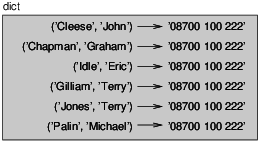Skip to main content

# 3.6: Dictionaries and Tuples

Dictionaries have a method called items that returns a list of tuples, where each tuple is a key-value pair.

>>> d = {'a':0, 'b':1, 'c':2}
>>> t = d.items()
>>> print t
[('a', 0), ('c', 2), ('b', 1)]


As you should expect from a dictionary, the items are in no particular order. In Python 3, items returns an iterator, but for many purposes, iterators behave like lists.

Going in the other direction, you can use a list of tuples to initialize a new dictionary:

>>> t = [('a', 0), ('c', 2), ('b', 1)]
>>> d = dict(t)
>>> print d
{'a': 0, 'c': 2, 'b': 1}


Combining dict with zip yields a concise way to create a dictionary:

>>> d = dict(zip('abc', range(3)))
>>> print d
{'a': 0, 'c': 2, 'b': 1}


The dictionary method update also takes a list of tuples and adds them, as key-value pairs, to an existing dictionary.

Combining items, tuple assignment and for, you get the idiom for traversing the keys and values of a dictionary:

for key, val in d.items():
print val, key


The output of this loop is:

0 a
2 c
1 b


Again.

It is common to use tuples as keys in dictionaries (primarily because you can’t use lists). For example, a telephone directory might map from last-name, first-name pairs to telephone numbers. Assuming that we have defined last, first and number, we could write:

directory[last,first] = number


The expression in brackets is a tuple. We could use tuple assignment to traverse this dictionary.

for last, first in directory:
print first, last, directory[last,first]


This loop traverses the keys in directory, which are tuples. It assigns the elements of each tuple to last and first, then prints the name and corresponding telephone number.

There are two ways to represent tuples in a state diagram. The more detailed version shows the indices and elements just as they appear in a list. For example, the tuple ('Cleese', 'John') would appear as in Figure 12.6.1.Figure $$\PageIndex{1}$$: State diagram.

But in a larger diagram you might want to leave out the details. For example, a diagram of the telephone directory might appear as in Figure 12.6.2.Figure $$\PageIndex{2}$$: State diagram.

Here the tuples are shown using Python syntax as a graphical shorthand.

The telephone number in the diagram is the complaints line for the BBC, so please don’t call it.

• Was this article helpful?